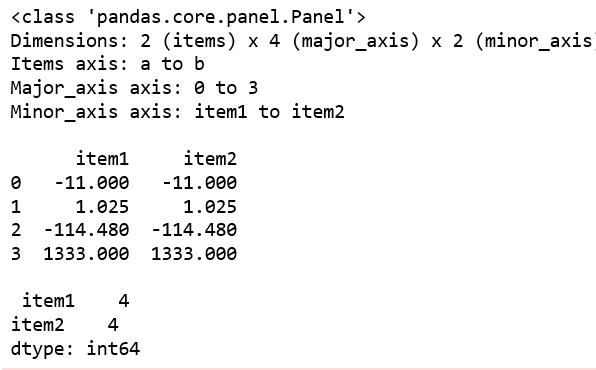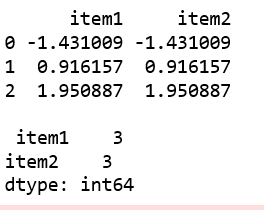Open in App
Not now

# Python | Pandas Panel.count()

• Last Updated : 01 Jan, 2019

In Pandas, Panel is a very important container for three-dimensional data. The names for the 3 axes are intended to give some semantic meaning to describing operations involving panel data and, in particular, econometric analysis of panel data.

`Panel.count()` function is used to return number of observations over requested axis.

Syntax: Panel.count(axis=’major’)

Parameters: axis : {‘items’, ‘major’, ‘minor’} or {0, 1, 2}

Returns: count of DataFrame

Code #1:

 `# importing pandas module ``import` `pandas as pd ``import` `numpy as np`` ` `df1 ``=` `pd.DataFrame({``'a'``: [``'Geeks'``, ``'For'``, ``'geeks'``, ``'real'``], ``                    ``'b'``: [``-``11``, ``+``1.025``, ``-``114.48``, ``1333``]})``                     ` `data ``=` `{``'item1'``:df1, ``'item2'``:df1}`` ` `# creating Panel ``panel ``=` `pd.Panel.from_dict(data, orient ``=``'minor'``)``print``(panel, ``"\n"``)``print``(panel[``'b'``])`` ` `print``(``"\n"``, panel[``'b'``].count())`

Output:Code #2:

 `# importing pandas module ``import` `pandas as pd ``import` `numpy as np`` ` `df1 ``=` `pd.DataFrame({``'a'``: [``'Geeks'``, ``'For'``, ``'geeks'``], ``                    ``'b'``: np.random.randn(``3``)})``                     ` `data ``=` `{``'item1'``:df1, ``'item2'``:df1}`` ` `# creating Panel ``panel ``=` `pd.Panel.from_dict(data, orient ``=``'minor'``)``print``(panel, ``"\n"``)``print``(panel[``'b'``])`` ` ` ` `df2 ``=` `pd.DataFrame({``'b'``: [``11``, ``12``, ``13``]})``print``(``"\n"``, panel[``'b'``].count())`

Output:My Personal Notes arrow_drop_up